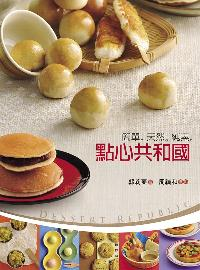### 點心共和國

• 點閱：1
• 並列題名：Dessert republic
• 作者：
• 出版年：2011[民100]
• 出版社：法鼓文化
• 出版地：臺北市
• ISBN：978-957-598-549-3 ; 957-598-549-4

##章節

• 封面(p.a)
• 空白(p.b)
• 扉頁(p.1)
• 自序：一圓點心夢(p.2)
• 自序：一圓點心夢(p.3)
• 自序：一圓點心夢(p.4)
• 自序：一圓點心夢(p.5)
• 目錄(p.6)
• 目錄(p.7)
• 傳奇的中國(p.8)
• 傳奇的中國(p.9)
• 香蕉發糕(p.10)
• 香蕉發糕(p.11)
• 雪花糕(p.12)
• 雪花糕(p.13)
• 絲瓜湯包(p.14)
• 絲瓜湯包(p.15)
• 水果淋餅(p.16)
• 燒賣(p.17)
• 水梨冰糖白木耳湯(p.18)
• 水梨冰糖白木耳湯(p.19)
• 老薑黑糖糕(p.20)
• 老薑黑糖糕(p.21)
• 五穀雜糧芋頭糕(p.22)
• 五穀雜糧芋頭糕(p.23)
• 甜美的日本(p.24)
• 甜美的日本(p.25)
• 番薯餅(p.26)
• 番薯餅(p.27)
• 銅鑼燒(p.28)
• 銅鑼燒(p.29)
• 關東饅頭(p.30)
• 關東饅頭(p.31)
• 五平燒(p.32)
• 五平燒(p.33)
• 薑汁熱麻糬(p.34)
• 薑汁熱麻糬(p.35)
• 亞麻子餅乾(p.36)
• 亞麻子餅乾(p.37)
• 神祕的印度(p.38)
• 神祕的印度(p.39)
• 三角餅(p.40)
• 三角餅(p.41)
• 咖哩醬烤餅(p.42)
• 咖哩醬烤餅(p.43)
• 糖漬水果(p.44)
• 糖漬水果(p.45)
• 甩餅(p.46)
• 甩餅(p.47)
• 香料球(p.48)
• 香料球(p.49)
• 清涼的南洋(p.50)
• 清涼的南洋(p.51)
• 香蕉酥(p.52)
• 香蕉酥(p.53)
• 玉米甜糕(p.54)
• 玉米甜糕(p.55)
• 西米番薯園(p.56)
• 西米番薯園(p.57)
• 鳳梨涼糕(p.58)
• 鳳梨涼糕(p.59)
• 鳳梨莎莎餅(p.60)
• 鳳梨莎莎餅(p.61)
• 幸福的西藏(p.62)
• 幸福的西藏(p.63)
• 蛻(p.64)
• 蛻(p.65)
• 甜麵(p.66)
• 甜麵(p.67)
• 糢糢(p.68)
• 糢糢(p.69)
• 安多鬆餅(p.70)
• 安多鬆餅(p.71)
• 黃金球(p.72)
• 黃金球(p.73)
• 巴勒(p.74)
• 巴勒(p.75)
• 豪邁的美國(p.76)
• 豪邁的美國(p.77)
• 甜甜圈(p.78)
• 甜甜圈(p.79)
• 焦糖爆米花(p.80)
• 焦糖爆米花(p.81)
• 鬆餅(p.82)
• 鬆餅(p.83)
• 焦糖蘋果派(p.84)
• 豆漿淡糕(p.85)
• 布朗尼(p.86)
• 布朗尼(p.87)
• 巧克力餅乾(p.88)
• 巧克力餅乾(p.89)
• 華麗的中東(p.90)
• 華麗的中東(p.91)
• 芝麻雙茄口袋餅(p.92)
• 芝麻雙茄口袋餅(p.93)
• 玫瑰杏仁糖(p.94)
• 玫瑰杏仁糖(p.95)
• 核果酥(p.96)
• 核果酥(p.97)
• 椰子餅(p.98)
• 椰子餅(p.99)
• 南瓜餃子(p.100)
• 南瓜餃子(p.101)
• 夢幻的歐洲(p.102)
• 夢幻的歐洲(p.103)
• 蔬菜脆薄餅(p.104)
• 蔬菜脆薄餅(p.105)
• 杏仁柳橙塔(p.106)
• 杏仁柳橙塔(p.107)
• 法國麵包(p.108)
• 法國麵包(p.109)
• 比利時南瓜湯(p.110)
• 比利時南瓜湯(p.111)
• 司康(p.112)
• 司康(p.113)
• 咖啡餅乾(p.114)
• 咖啡餅乾(p.115)
• 藍莓貝果(p.116)
• 藍莓貝果(p.117)
• 馬德蓮(p.118)
• 馬德蓮(p.119)
• 版權(p.a)
• 空白(p.b)
• 封底(p.c)